# 96.不同的二叉搜索树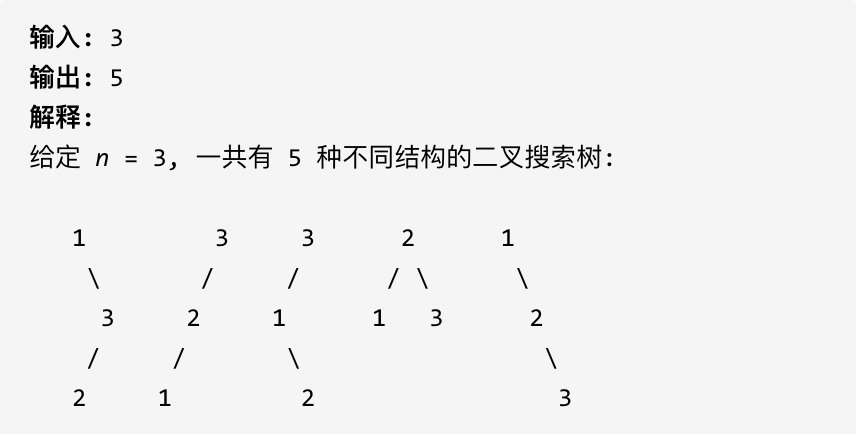# 思路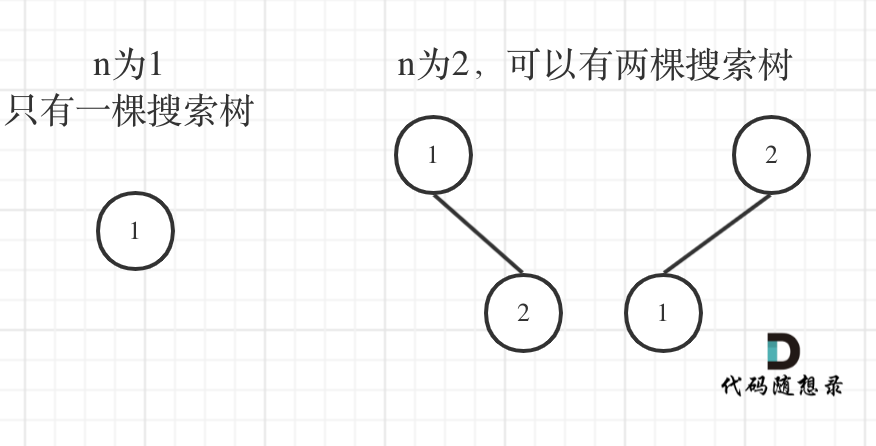n为1的时候有一棵树，n为2有两棵树，这个是很直观的。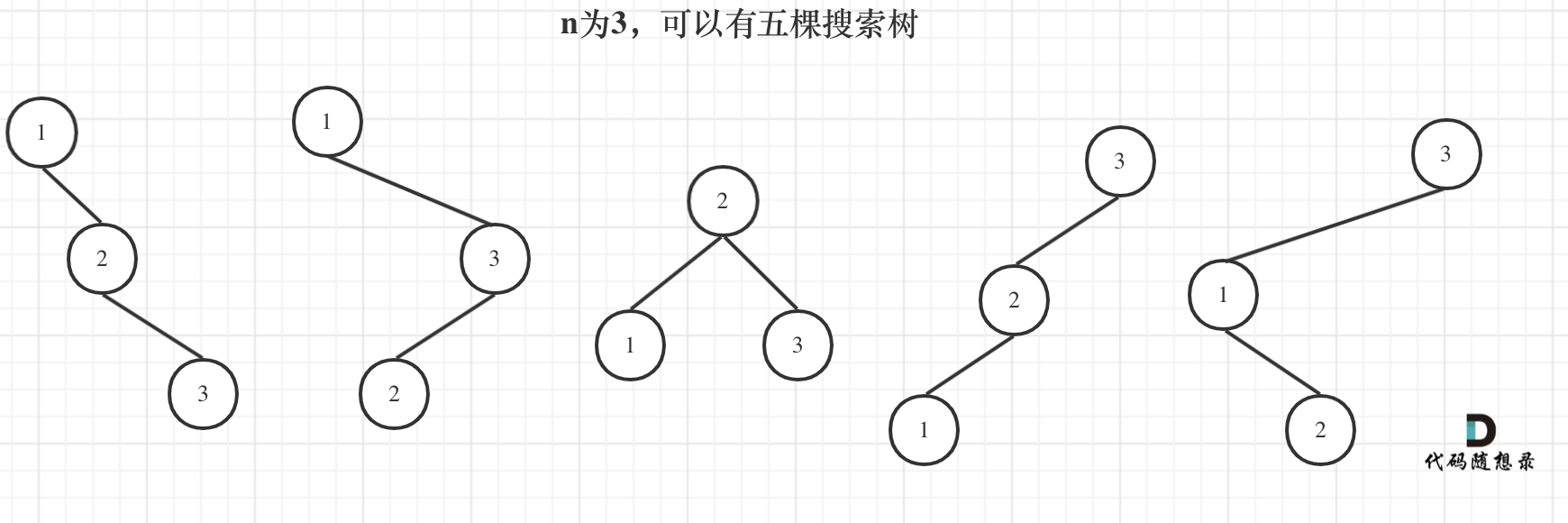（可能有同学问了，这布局不一样啊，节点数值都不一样。别忘了我们就是求不同树的数量，并不用把搜索树都列出来，所以不用关心其具体数值的差异）

dp，就是 元素1为头结点搜索树的数量 + 元素2为头结点搜索树的数量 + 元素3为头结点搜索树的数量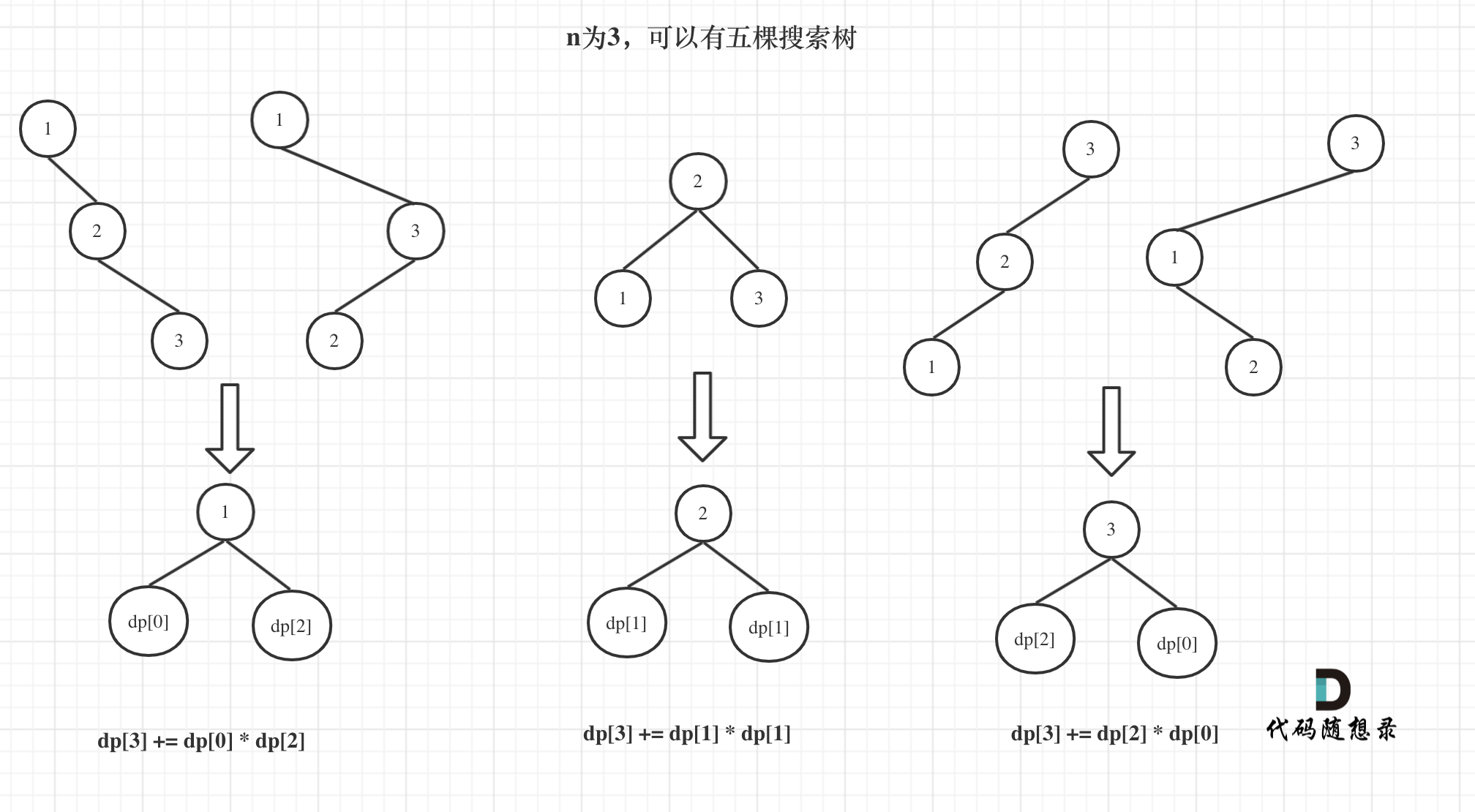1. 确定dp数组（dp table）以及下标的含义

dp[i] ： 1到i为节点组成的二叉搜索树的个数为dp[i]

1. 确定递推公式

j相当于是头结点的元素，从1遍历到i为止。

1. dp数组如何初始化

1. 确定遍历顺序

for (int i = 1; i <= n; i++) {
for (int j = 1; j <= i; j++) {
dp[i] += dp[j - 1] * dp[i - j];
}
}
1
2
3
4
5
1. 举例推导dp数组

n为5时候的dp数组状态如图：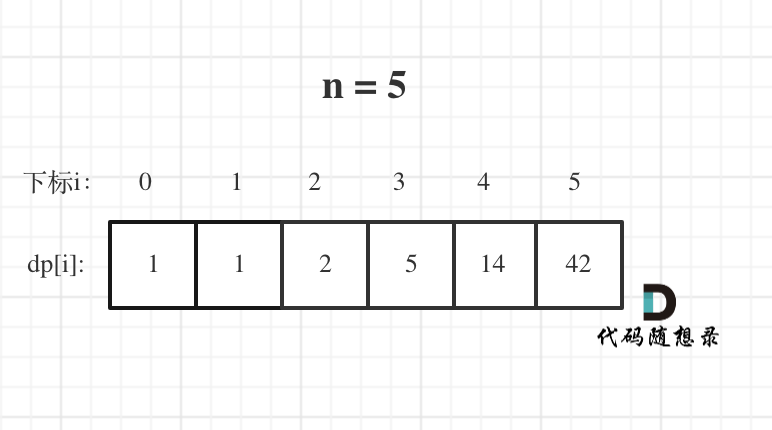class Solution {
public:
int numTrees(int n) {
vector<int> dp(n + 1);
dp = 1;
for (int i = 1; i <= n; i++) {
for (int j = 1; j <= i; j++) {
dp[i] += dp[j - 1] * dp[i - j];
}
}
return dp[n];
}
};
1
2
3
4
5
6
7
8
9
10
11
12
13
• 时间复杂度：\$O(n^2)\$
• 空间复杂度：\$O(n)\$

# 其他语言版本

# Java

class Solution {
public int numTrees(int n) {
//初始化 dp 数组
int[] dp = new int[n + 1];
//初始化0个节点和1个节点的情况
dp = 1;
dp = 1;
for (int i = 2; i <= n; i++) {
for (int j = 1; j <= i; j++) {
//对于第i个节点，需要考虑1作为根节点直到i作为根节点的情况，所以需要累加
//一共i个节点，对于根节点j时,左子树的节点个数为j-1，右子树的节点个数为i-j
dp[i] += dp[j - 1] * dp[i - j];
}
}
return dp[n];
}
}
1
2
3
4
5
6
7
8
9
10
11
12
13
14
15
16
17

# Python

class Solution:
def numTrees(self, n: int) -> int:
dp =  * (n + 1)
dp, dp = 1, 1
for i in range(2, n + 1):
for j in range(1, i + 1):
dp[i] += dp[j - 1] * dp[i - j]
return dp[-1]
1
2
3
4
5
6
7
8

# Go

func numTrees(n int)int{
dp:=make([]int,n+1)
dp=1
for i:=1;i<=n;i++{
for j:=1;j<=i;j++{
dp[i]+=dp[j-1]*dp[i-j]
}
}
return dp[n]
}
1
2
3
4
5
6
7
8
9
10

# Javascript

const numTrees =(n) => {
let dp = new Array(n+1).fill(0);
dp = 1;
dp = 1;

for(let i = 2; i <= n; i++) {
for(let j = 1; j <= i; j++) {
dp[i] += dp[j-1] * dp[i-j];
}
}

return dp[n];
};
1
2
3
4
5
6
7
8
9
10
11
12
13@2021-2022 代码随想录 版权所有 粤ICP备19156078号-3